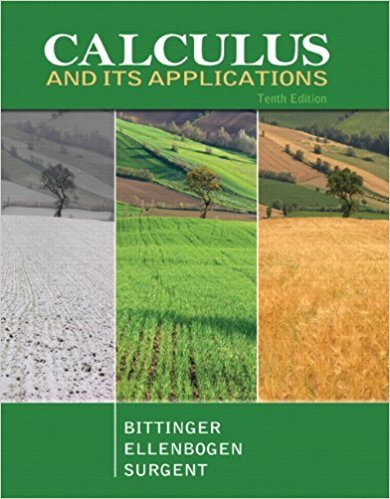×
×

# Solutions for Chapter 2.2: Using Second Derivatives to Find Maximum and Minimum Values and Sketch Graphs## Full solutions for Calculus and Its Applications | 10th Edition

ISBN: 9780321694331Solutions for Chapter 2.2: Using Second Derivatives to Find Maximum and Minimum Values and Sketch Graphs

Solutions for Chapter 2.2
4 5 0 252 Reviews
10
1
##### ISBN: 9780321694331

This expansive textbook survival guide covers the following chapters and their solutions. Calculus and Its Applications was written by and is associated to the ISBN: 9780321694331. This textbook survival guide was created for the textbook: Calculus and Its Applications, edition: 10. Since 128 problems in chapter 2.2: Using Second Derivatives to Find Maximum and Minimum Values and Sketch Graphs have been answered, more than 24907 students have viewed full step-by-step solutions from this chapter. Chapter 2.2: Using Second Derivatives to Find Maximum and Minimum Values and Sketch Graphs includes 128 full step-by-step solutions.

Key Calculus Terms and definitions covered in this textbook
• Angle

Union of two rays with a common endpoint (the vertex). The beginning ray (the initial side) can be rotated about its endpoint to obtain the final position (the terminal side)

• Arc length formula

The length of an arc in a circle of radius r intercepted by a central angle of u radians is s = r u.

• Arccosecant function

See Inverse cosecant function.

• Axis of symmetry

See Line of symmetry.

• Constant term

See Polynomial function

• Derivative of ƒ

The function defined by ƒ'(x) = limh:0ƒ(x + h) - ƒ(x)h for all of x where the limit exists

• Double inequality

A statement that describes a bounded interval, such as 3 ? x < 5

• Inverse cosecant function

The function y = csc-1 x

• Mapping

A function viewed as a mapping of the elements of the domain onto the elements of the range

• Maximum r-value

The value of |r| at the point on the graph of a polar equation that has the maximum distance from the pole

• Measure of center

A measure of the typical, middle, or average value for a data set

• Modulus

See Absolute value of a complex number.

• Negative association

A relationship between two variables in which higher values of one variable are generally associated with lower values of the other variable.

• Normal distribution

A distribution of data shaped like the normal curve.

• Partial fraction decomposition

See Partial fractions.

• Reflection across the x-axis

x, y and (x,-y) are reflections of each other across the x-axis.

• Sequence

See Finite sequence, Infinite sequence.

• Sinusoid

A function that can be written in the form f(x) = a sin (b (x - h)) + k or f(x) = a cos (b(x - h)) + k. The number a is the amplitude, and the number h is the phase shift.

• Sum of an infinite geometric series

Sn = a 1 - r , |r| 6 1